Examples

Chapter 11 Class 12 Three Dimensional Geometry
Serial order wise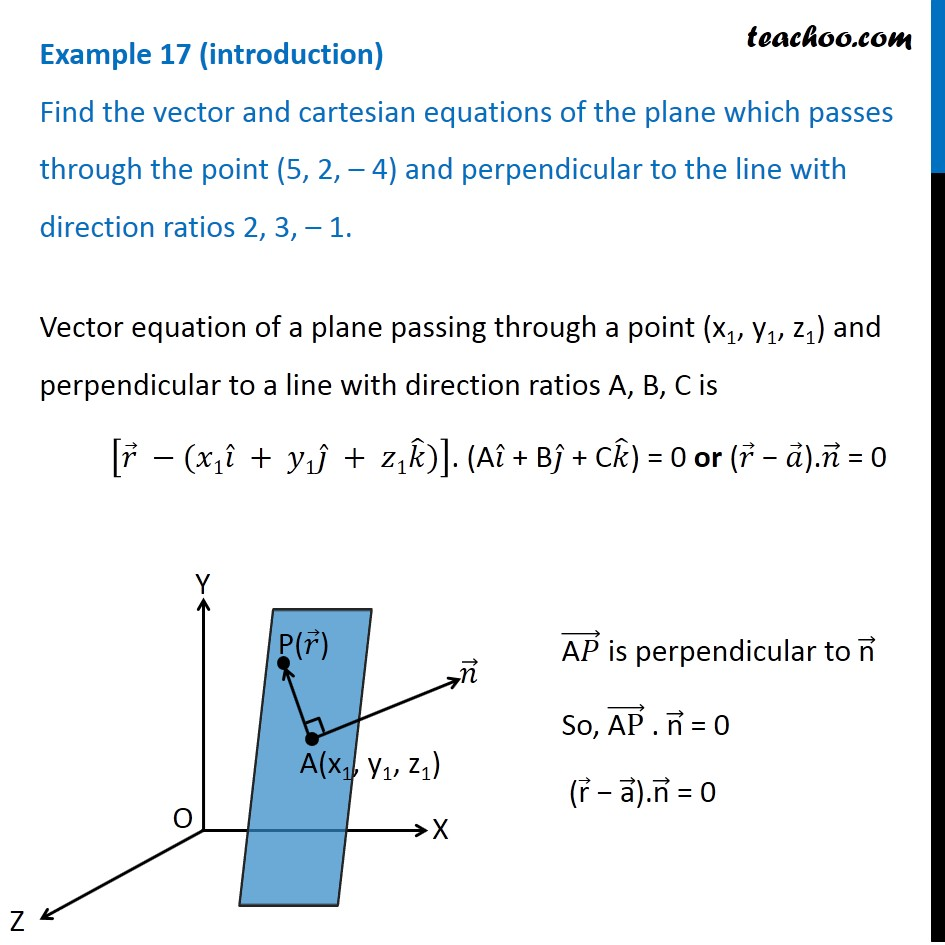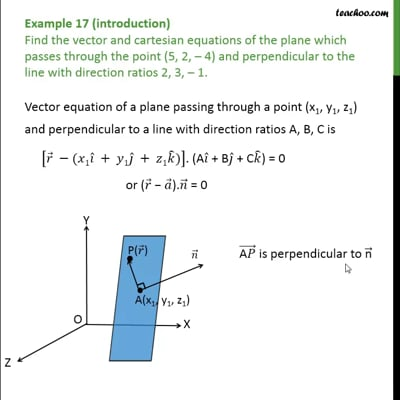This video is only available for Teachoo black users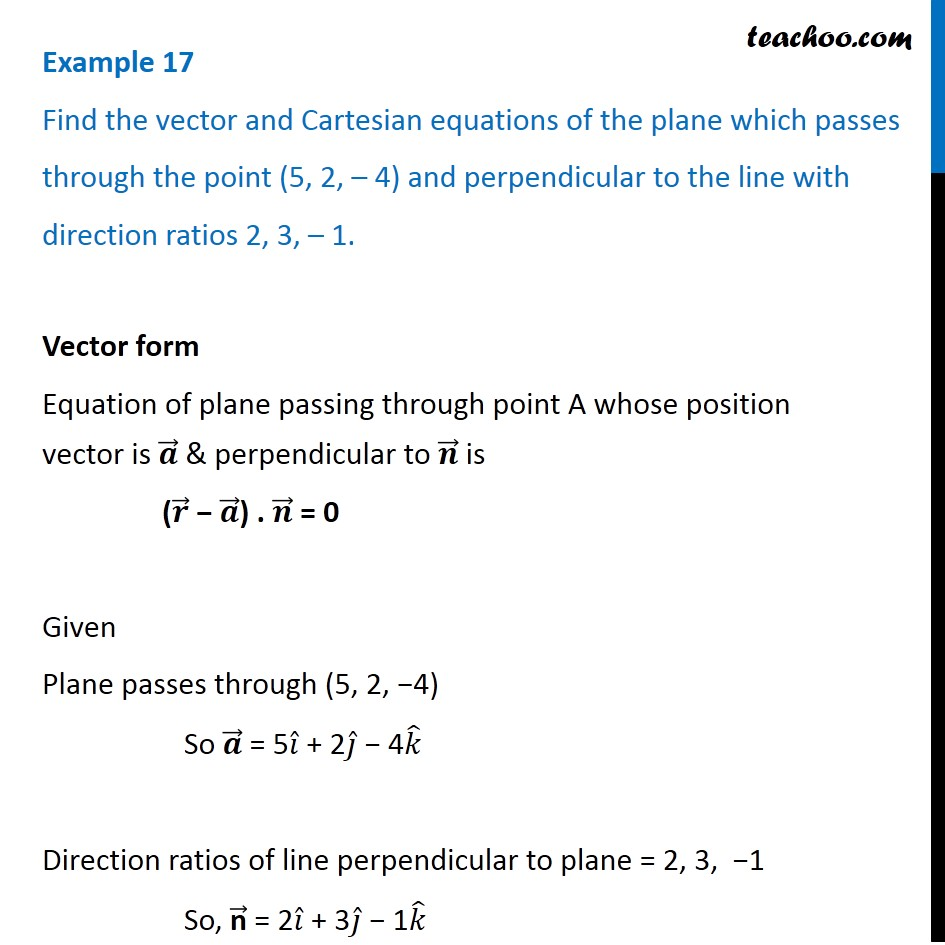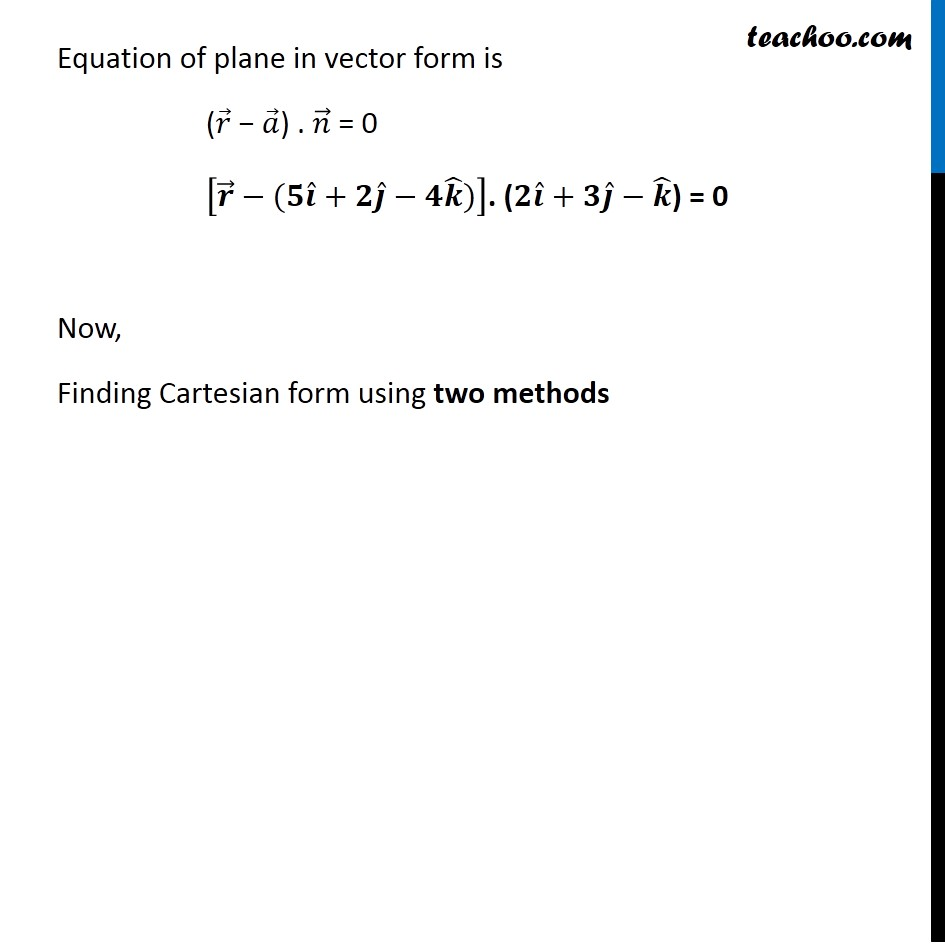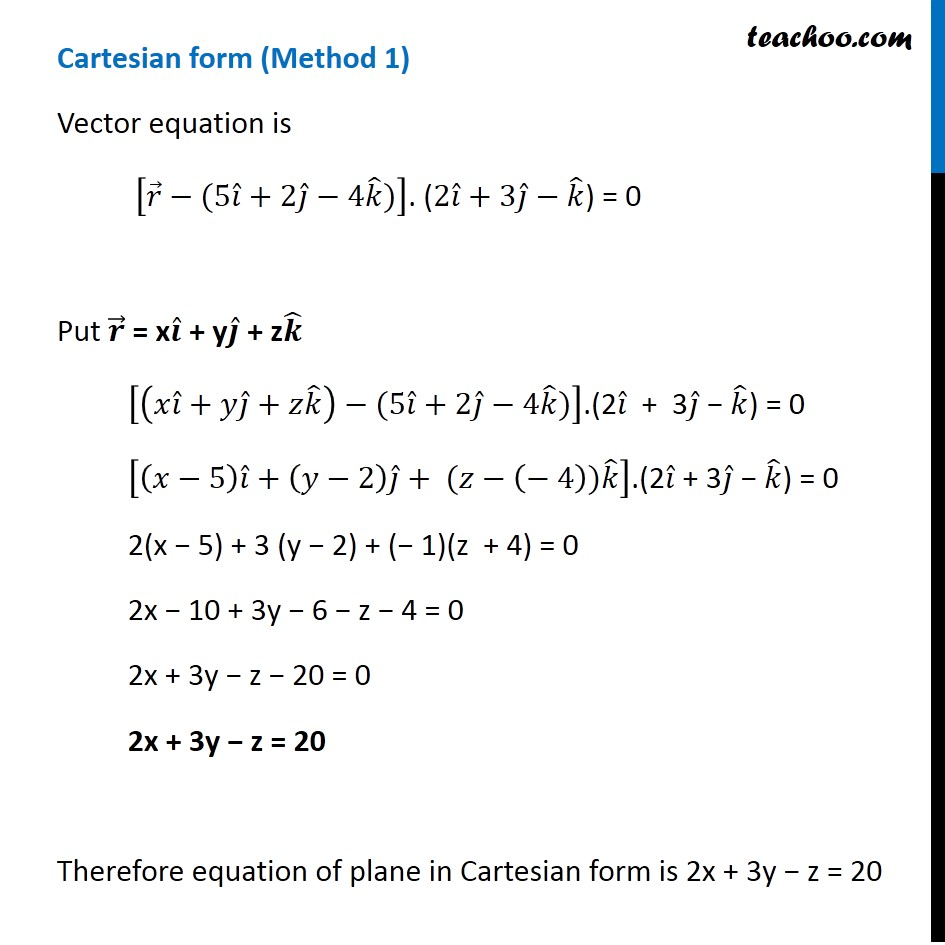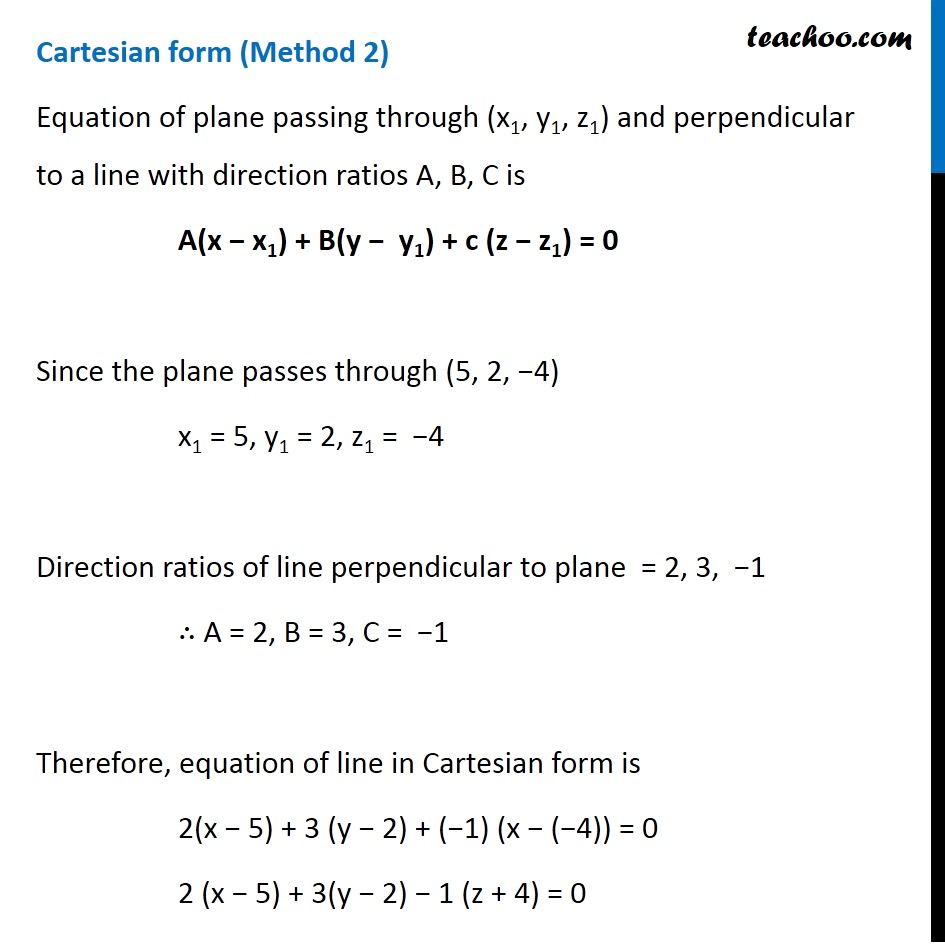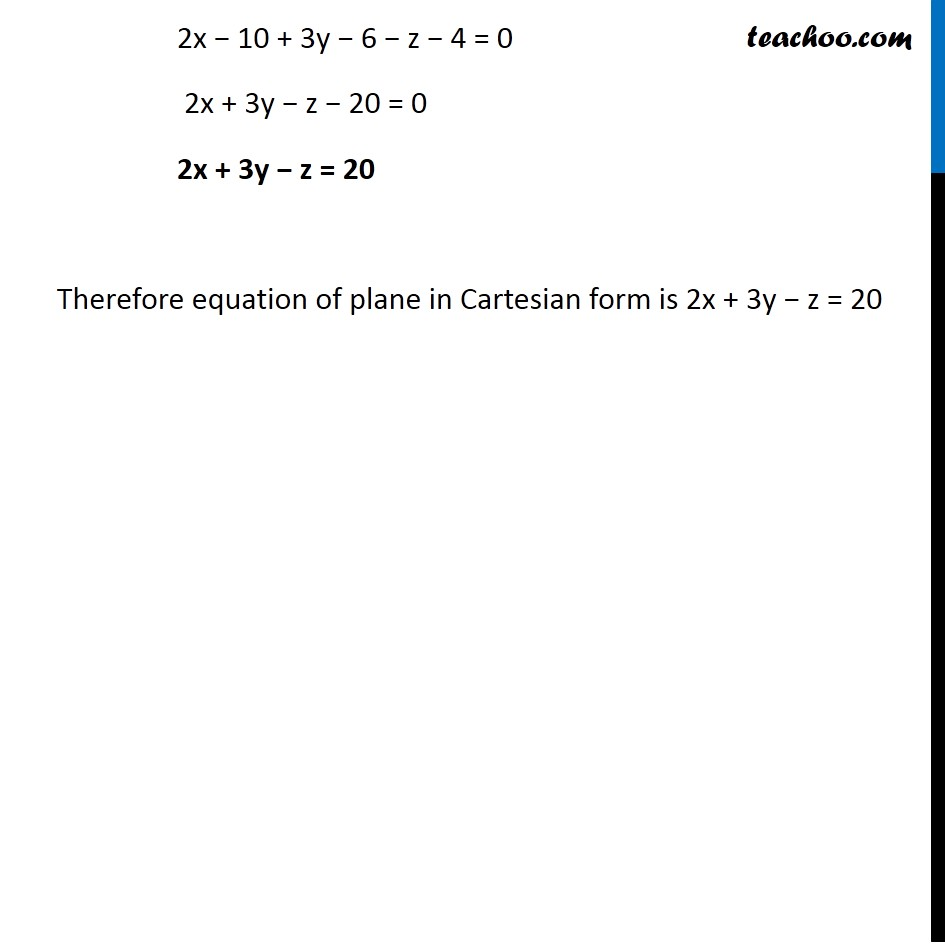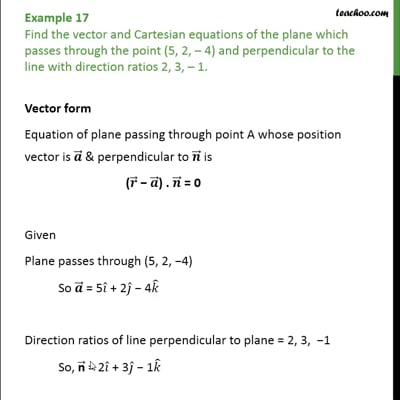This video is only available for Teachoo black users

Get live Maths 1-on-1 Classs - Class 6 to 12

### Transcript

Example 17 (introduction) Find the vector and cartesian equations of the plane which passes through the point (5, 2, – 4) and perpendicular to the line with direction ratios 2, 3, – 1.Vector equation of a plane passing through a point (x1, y1, z1) and perpendicular to a line with direction ratios A, B, C is [𝑟 ⃗ −(𝑥1𝑖 ̂ + 𝑦1𝑗 ̂ + 𝑧1𝑘 ̂)]. (A𝑖 ̂ + B𝑗 ̂ + C𝑘 ̂) = 0 or (𝑟 ⃗ − 𝑎 ⃗).𝑛 ⃗ = 0 ("A" 𝑃) ⃗ is perpendicular to "n" ⃗ So, ("A" P) ⃗ . "n" ⃗ = 0 ("r" ⃗ − "a" ⃗)."n" ⃗ = 0 Example 17 Find the vector and Cartesian equations of the plane which passes through the point (5, 2, – 4) and perpendicular to the line with direction ratios 2, 3, – 1.Vector form Equation of plane passing through point A whose position vector is 𝒂 ⃗ & perpendicular to 𝒏 ⃗ is (𝒓 ⃗ − 𝒂 ⃗) . 𝒏 ⃗ = 0 Given Plane passes through (5, 2, −4) So 𝒂 ⃗ = 5𝑖 ̂ + 2𝑗 ̂ − 4𝑘 ̂ Direction ratios of line perpendicular to plane = 2, 3, −1 So, "n" ⃗ = 2𝑖 ̂ + 3𝑗 ̂ − 1𝑘 ̂ Equation of plane in vector form is (𝑟 ⃗ − 𝑎 ⃗) . 𝑛 ⃗ = 0 [𝒓 ⃗−(𝟓𝒊 ̂+𝟐𝒋 ̂−𝟒𝒌 ̂)]. (𝟐𝒊 ̂+𝟑𝒋 ̂−𝒌 ̂) = 0 Now, Finding Cartesian form using two methods Cartesian form (Method 1) Vector equation is [𝑟 ⃗−(5𝑖 ̂+2𝑗 ̂−4𝑘 ̂)]. (2𝑖 ̂+3𝑗 ̂−𝑘 ̂) = 0 Put 𝒓 ⃗ = x𝒊 ̂ + y𝒋 ̂ + z𝒌 ̂ [(𝑥𝑖 ̂+𝑦𝑗 ̂+𝑧𝑘 ̂ )−(5𝑖 ̂+2𝑗 ̂−4𝑘 ̂)].(2𝑖 ̂ + 3𝑗 ̂ − 𝑘 ̂) = 0 [(𝑥−5) 𝑖 ̂+(𝑦−2) 𝑗 ̂+ (𝑧−(− 4))𝑘 ̂ ].(2𝑖 ̂ + 3𝑗 ̂ − 𝑘 ̂) = 0 2(x − 5) + 3 (y − 2) + (− 1)(z + 4) = 0 2x − 10 + 3y − 6 − z − 4 = 0 2x + 3y − z − 20 = 0 2x + 3y − z = 20 Therefore equation of plane in Cartesian form is 2x + 3y − z = 20 Cartesian form (Method 2) Equation of plane passing through (x1, y1, z1) and perpendicular to a line with direction ratios A, B, C is A(x − x1) + B(y − y1) + c (z − z1) = 0 Since the plane passes through (5, 2, −4) x1 = 5, y1 = 2, z1 = −4 Direction ratios of line perpendicular to plane = 2, 3, −1 ∴ A = 2, B = 3, C = −1 Therefore, equation of line in Cartesian form is 2(x − 5) + 3 (y − 2) + (−1) (x − (−4)) = 0 2 (x − 5) + 3(y − 2) − 1 (z + 4) = 0 2x − 10 + 3y − 6 − z − 4 = 0 2x + 3y − z − 20 = 0 2x + 3y − z = 20 Therefore equation of plane in Cartesian form is 2x + 3y − z = 20